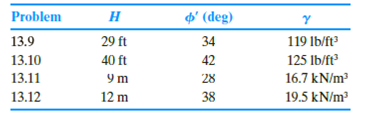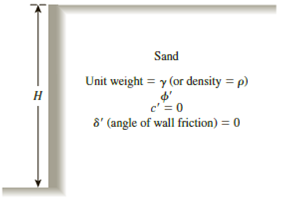Chapter 13, Problem 13.10PPrinciples of Geotechnical Enginee...

9th Edition
Braja M. Das + 1 other
ISBN: 9781305970939

Solutions

Chapter
SectionPrinciples of Geotechnical Enginee...

9th Edition
Braja M. Das + 1 other
ISBN: 9781305970939
Textbook Problem

13.9 through 13.12 Assume that the retaining wall shown in Figure 13.39 is frictionless. For each problem, determine the Rankine active force per unit length of the wall, the variation of active earth pressure with depth, and the location of the resultant.Figure 13.39

To determine

The Rankine active force σa(z=H) per unit length of the wall, the variation of active earth pressure Pa with depth, and the location z¯ of the resultant measured from the bottom of the wall.

Explanation

Given information:

The height (H) of the retaining wall is 40 ft.

The soil friction angle ϕ is 42°.

The angle of wall friction δ is 0.

The unit weight γ of the soil is 125lb/ft3.

Calculation:

Calculate the active earth pressure coefficient (Ka) using the relation.

Ka=tan2(45ϕ2)

Substitute 42° for ϕ.

Ka=tan2(4542°2)=tan2(4521)=tan2(24)=0.198

Calculate Rankine active force σa(z=H) per unit length of the wall using the relation.

σa(z=H)=KaγH

Substitute 0.198 for Ka, 125lb/ft3 for γ, and 40 ft for H.

σa(z=H)=0

Still sussing out bartleby?

Check out a sample textbook solution.

See a sample solution

The Solution to Your Study Problems

Bartleby provides explanations to thousands of textbook problems written by our experts, many with advanced degrees!

Get Started

What are the most popular encryption systems used over the Web?

Principles of Information Security (MindTap Course List)

Explain the differences among data, information, and a database.

Database Systems: Design, Implementation, & Management

How would you explode DFDs?

Systems Analysis and Design (Shelly Cashman Series) (MindTap Course List)

Name three popular welding processes.

Welding: Principles and Applications (MindTap Course List)